Friday, September 27, 2019

Methods of resolution of the improper integrals

Objective: Solve the different types of improper integrals

Definition. An improper integral is an integral  where one or  the two limits of integration are infinite. An integral is also improper when the function has an infinite discontinuity. Let's look at the two scenarios:

I. One or two of the limits of integration are infinite.

a. One of the limit of integration is finite,

If f is continuous over the interval [a, +∞[, then
If the limit is finite the integral converges. If the limit is infinite the integral diverges.
Geometric Interpretation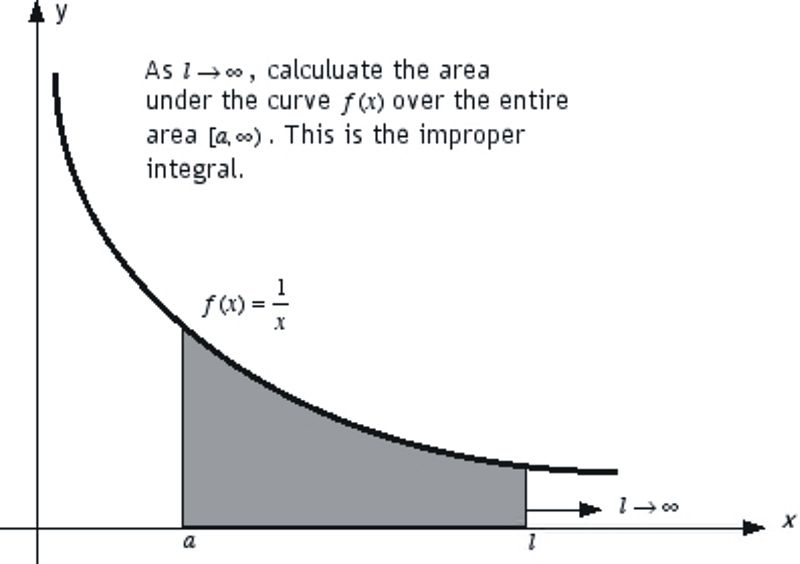Let's consider a function f(x) = 1/x. The integral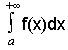represents the area under the curve of the function that starts from the vertical passing by a and extending to infinity.
The integralrepresents the area between the verticals passing by a and l. As l approaches infinity, the area under the curve spreads to infinity. It's fair to say that this situation represents.
We can defineas the limit ofwhen l approaches infinity.
We can then set a rule to calculate the improper integral where the lower limit is finite and the upper limit is infinite. In this case we have to replace the infinite limit by l and find the limit ofwhen l approaches infinity.

Example 1. Calculate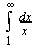Solution

Let's replace infinity by l and calculate the limit of the finite integral when l approaches infinity.

We have:
b. The two limits of integration are  infinite

In this case we choose any value a in the interval ]-∞, +∞[ and calculate the integral over the sub-intervals
]-∞, a[∪ ]a ,  +∞[

Solution

Let's split the integral over the intervasl ]-,0] and [0,+[: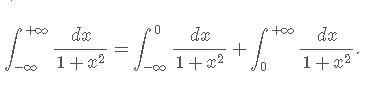Let's evaluate the first integral on the right side: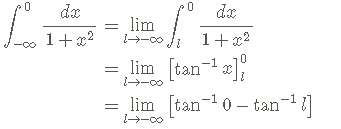Let's calculate the limit by substituting l by -∞:

Let's evaluate the second integral:

Let's substitute the two integrals we obtain:

II The function to integrate is discontinued.

Let's say that we have to calculate an integral over an interval ]a, b[ where the function f is continued. If the function isn't continued at a point c of the interval, we evaluate the integral over the intervals ]a,c[∪]c,d[.

Example. Evaluate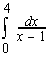Solution

The function is discontinued at x = 1. Therefore we integrate it over the 2 intervals ]0,1[∪ ]1.4[.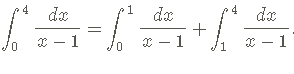Let's calculate the first integral: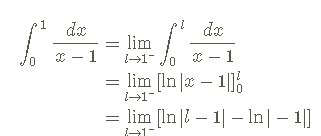= ln │1⁻-1│-ln1
= ln o⁺
= -∞

Since the first integral diverges there's no reason to calculate the second integral. The given integral diverges.

Exercises
Evaluate. Tell if the integral converges or diverges:

If you want to learn more about Calculus check this site Center for Integral Development
.

Friday, May 31, 2019

The definite integral and the integration by substitution

Objective:

Apply the method of integration by substitution to the resolution of definite integrals

Method (Recall)

The method by substitution consists in substituting the given variable by another variable
that makes the integral easier to calculate.

Let’s integrateby substitution.

If this integral can be writtenwithandthenThe integralbecomes easier to calculate. In the case of a definite integral
we have to calculate the new limits of integration for u.

Example I

Let’s calculate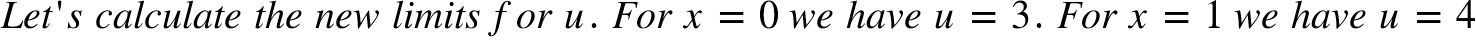======Example II EvaluateWe can rewrite the expression as:

We have here 2 separate expressionsand x²

We seek to find out if the expression closed to dx is the derivative of the expression x³ in.

We notice that is almost the derivative of . It misses the term 3. Therefore we have
to multiply and divide by 3 in order to have 3 . The given expression becomes: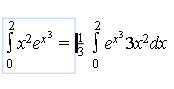represents a function composite of the exponential function f(x) =and the function g(x) =

We can write=  f(g(x)). 3x represents the derivative of x³. We can write   3x².= . g'(x)

Then we have:

If we do a change of variable by writing u = x³ du/dx = 3x² du = 3x²dx.= g'(x)dx

f(g(x)) =. becomes f(u) = .. There are new limits of integration for the new function of u

In the expresion u = x³, for x = 0 u = 0, for x = 2 u = (2)³ = 8

Let's substitute f(g(x)) by f(u) and g'(x)dx by du:

Let's substitute f(u) by: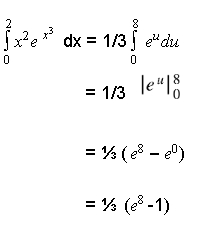N.B. We have solved the second example by writing the given function as a composite function. We can skip these steps by doing the change of variable right away.as it was done in the first example,

Practice

Thursday, May 2, 2019

The definite integral and its properties

Objectives:

1. Define the definite integral
2. Evaluate the definite integral
3. List the properties of definite integrals

Introduction

Referring to the post: An approach to calculate the area under curve: the definite integral, we define the area under the curve between x₀ and x₄ by:

This expression represents the integral of the function between  x₀ and x₄. We can replace these values by a and b.We have :

Method to evaluate definite integrals

We are going to use a practical method discovered by Newton to evaluate definite integrals. This method uses antiderivatives for the evaluation of definite integrals.

Theorem

Properties of definite integrals

Examples

Solve the definite integrals:

Solutions

Practice. Use antiderivatives to calculate the following definite integrals:

Interested in learning more about definite integrals visit Center for Integral Development

Friday, April 5, 2019

Integrating an expression with radical by using trigonometric substitutions

In order to integrate integrals containing the radical expressions ⎷a²-x², ⎷a² + x², ⎷x² - a², we put these expressions in trigonometric form. In order to find the equivalent trigonometric expressions, we make each of the expressions the side of a right triangle.

We start by drawing three right triangles whose  one side is one of these expressions : ⎷a²-x², ⎷a² + x², ⎷x² - a²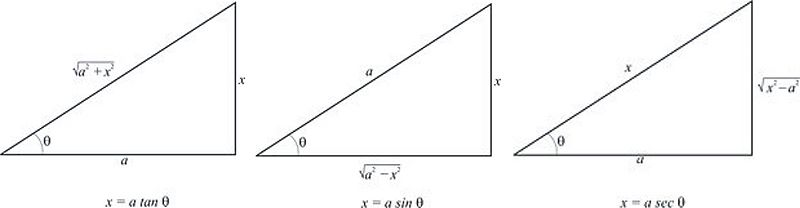Case 1 ⎷a²-x²

In this case we consider the middle triangle whose hypotenuse is a and the vertical side of the right angle is x. The horizontal side of the right angle is the expression containing the radical.

In considering the relations in a right triangle we write x = asinθ. In this case we use the identity 1-sin²θ = cos²θ

Case 2 ⎷a² + x²

In this case we consider the first right triangle whose hypotenuse is  ⎷a² + x²

In a right triangle the tangent is equal to the ratio of the opposite side to the adjacent side. Therefore tanθ = x/a then x = atanθ. We use the identity  1 + tan² = sec²θ

Case 3 ⎷x² - a²

We consider the third triangle where the vertical side of the right angle is ⎷x² - a². In a right triangle a side of a right angle is equal to the product of the hypotenuse by the cosine of the adjacent acute angle. Therefore  a = xcosθ then x = a/cosθ x = a.1/cosθ x= asecθ the relation used is sec²θ-1 = tan²θ

Example I Evaluate ∫dx/x²⎷4-x².

Pay attention to the fraction bar. Read  ∫dx over x²⎷4-x². The radical affects the whole expression 4-x². The software I am using doesn't give me the horizontal bar of the radical sign.

Here we have case 1 ⎷a²-x². We have ⎷4-x² = √2² - x² then   a = 2. Therefore x = asinθ  x = 2sinθ. The identity used is  1-sin²θ = cos²θ . For x = asinθ we have dx/dA = 2cosθ. Then dx = 2cosθdθ.

Let's transform x²⎷4-x²:

x²⎷4-x² = 4sin²θ√4-4 4sin²θ

=  4sin²θ√4(1-sin²θ)

=  4sin²θ√4cos²θ

=   4sin²θ2cosθ

=   8sin²θcosθ

Let's substitute dx and x²⎷4-x in the original expression. we have;

∫dx/x²⎷4-x² = ∫2cosθdθ/ 8sin²θcosθ

=  ∫dθ/ 4sin²θ

= 1/4∫1/sin²θdθ

=1/4∫1/csc²θdθ
= -1/4cotθ + C

Let's express cotθ in function of x. Using the right triangle we can write: tanθ = x/ ⎷a²-x². then cotθ =   ⎷a²-x²/x

Let's substitute a by 2 we have  tanθ = x/ ⎷4-x². Then cotθ = ⎷4-x²/x.

Finally we have ∫dx/x²⎷4-x² = -1/4 ⎷4-x²/x + C

Example2  Evaluate ∫√x²-3/x dx

Here we have ⎷a²-x² we use the relation x = asecθ
Let's find a:

√x²-3 = √x²-(√3)². Let's note that the radical affect x²-(√3)². We have a = √3. then x = √3secA

dx = √3tanθsecθdθ

Let's substitute x and dx:

∫√x²-3/x dx = ∫√(√3secθ)²-3/√3secθ.√3tanθsecθdθ

= ∫√3sec²θ-3/√3secθ.√3tanθsecθdθ

= ∫√3sec²θ-3/√3secθ.√3secθtanθdθ (rearranging the expression to have √3secθ)

=   ∫√3sec²θ-3tanθdθ (simplifying by √3secθ)

=   ∫⇃3(sec²θ-1)tanθdθ

=   ∫√3 tan²θtanθdθ

=    ∫√3 tanθtanθdθ

=     ∫√3tan²θdθ

Let's calculate  ∫√3tan²θdθ

Let's apply the formula ∫tanⁿx =  tanⁿ⁻¹x/n-1 - ∫tanⁿ⁻²xdx

∫√3tan²θdθ = √3∫tan²θdθ

=  √3(tanθ -∫dθ)

=   √3(tanθ - θ) + C

Let's calculate tan using the third rectangle triangle:
tanθ = √x² - a²/a = √x²-3/√3. We use the rule that in a right triangle the tangent of an acute angle is equal to the ratio of the opposite side to the adjacent side. We can also say that A = tan⁻¹( √x²-3/√3)
∫√x²-3/x dx =  √3[√x²-3/√3 - tan⁻¹(√x²-3/√3)] + C
=  √x²-3 - √3 tan⁻¹(√x²-3/√3) + C

Practice   Evaluate ∫ dx/x²√x² + 1

Interested in taking online Calculus courses and tutoring visit Center for Integral Development

Friday, March 15, 2019

Integrating the product of the power of secant by the power of tangent

Method

We consider the following cases:

1) n even let u = tanx and use the following identity: sec²x = tan²x + 1
2) m odd  u = secx  tan²x = sec²x -1
2) m even n odd reduce power of secx: tan²x = sec²x -1

Example I

Calculate ∫tan²xsec⁴xdx

sec⁴x is the near derivative of tan²x and n is even we write u = tanx.

Taking the derivative of both sides we have du = sec²xdx. dx = du/sec²x.

Let's substitute tanx and dx

∫tan²xsec⁴xdx = ∫u²sec⁴x.du/sec²x

=  ∫u²sec²xdu

We use the identity sec²x = tan²x + 1 in order to have the integral as a function of u. Then by substituting tanx by u: sec²x = u² + 1. Therefore:

∫tan²xsec⁴xdx =  ∫u²( u² + 1)du

=  ∫(u⁴ + u²)du

= u⁵/5 + u³ + C

= 1/5tan⁵x + 1/3tan³x + C

Example II

Solve ∫tan³xsec³xdx.

We start by looking at the condition for n. Here n is odd. There isn't a condition for n only. We look at the condition for m. Here m is odd then we write u = secx and tan²x = sec²x-1

Let's write u = secx. Then du = tanxsecxdx. Therefore dx = du/tanxsecx.

Let's substitute secx and dx.

∫tan³xsec³xdx = ∫tan³xu³.du/tanxu

=  ∫tan²xu²du
Let's express tanx in function of secx so that we can have the integral as a function of u

∫tan³xsec³xdx =  ∫(sec²x - 1)u²du

=  ∫(u² - 1)u²du

=   ∫u⁴du -  ∫u²du

= 1/5u⁵ - 1/3u³ + C

=  1/5sec⁵x -  1/3sec³x + C

Practice

Solve:

1) ∫tan²xsec³x
2) ∫tan³xsec⁴x

Interested in learning more about integrals visit Center for Integral Development
Intrested in private tutoring visit New Direction Education Services for contact information.

Monday, March 4, 2019

Integrating the power of secants and tangents

In this post you are going to learn how to integrate the power of secant and tangent. There are some formulas that allow to calculate these powers. Before to learn these formulas let's learn first the formulas to integrate the secant and tangent.

Formulas to integrate the function tangent and secant

You learn first the expression of tangent since if you know its integral it is easy to know the integral of secant. The integral of each of these functions has the function ln, sec.

The integral of tangent is an expression of secant

∫tanx = ln❘secx❘ + C

The integral of secant is an expression of sec and tangent. We just add tangent between the absolute bars.from the integral of tanx

∫secx = ln❘secx + tanx❘ + C

N.B. These formulas can be demonstrated but the demonstration is not done here.

Formulas to integrate the power of tangent and secant

Integral of the power of secant

The integral of the power of secant is made of two terms. The first term is a quotient. The second term is the product of a constant by the integral of secant. We are going to learn the formula according to the following steps:

1. The numerator of the quotient and the and the second part of the integral are the same. It is obtained by decreasing the exponent of secx by 2.

∫secⁿ x = secⁿ⁻²x +  ∫secⁿ⁻²x

2. The denominator of the first quotient is obtained by taking only the exponent n and decreasing it by 1

∫secⁿ x = secⁿ⁻²x/n-1 +  ∫secⁿ⁻²x

3. In the first term we take the quotient of the exponent (n-2) of secx and the denominator (n-1). We then obtain the quotient before the integral

∫secⁿ x = secⁿ⁻²x/n-1 + n-2/n-1  ∫secⁿ⁻²x. This is the formula of the integral of the power of secant. We just learn how to memorize  the formula.

Example. Calculate the integral  ∫sec³x

We apply the formula ∫secⁿ x = secⁿ⁻²x/n-1 + n-2/n-1  ∫secⁿ⁻²x.

We substitute n by 3:

∫sec³x =  sec³⁻²x/3-1 + 3-2/3-1  ∫sec³⁻²x.

=   secx/2 + 1/2 ∫secx

=  1/2secx +ln ❘secx + tanx❘ + C

Integral of the power of tangent

The integral of the power of tangent is given by the formula: ∫ tanⁿxdx = tanⁿ⁻ⁱx/n-1- ∫tanⁿ⁻²xdx

Example. Solve ∫tan⁵xdx

∫tan⁵xdx = tan⁴x/4 - ∫ tan³xdx

Let's calculate ∫ tan³xdx:

∫ tan³xdx = tan²x/2 - ∫ tanxdx

=    tan²x/2 - ln|secx| + C
=    tan²x/2 + ln|cosx| + C

Let's substitute  tan³xdx:

∫tan⁵xdx = tan⁴x/4 - tan²x/2 - ln|cosx| + C

Practice

Solve:

1) ∫ sec⁵xdx
2)  ∫tan³xdx

Interested in learning more about Calculus visit Center for Integral Development
For private tutoring visit New Direction Education Services

Friday, February 22, 2019

Integrating the product of the power of sine by the power of cosine

Integrating a function of the product of sine by cosine: f(x) = sin^mxcos^nx ( read sine  exponent m x by cos exponent n x)

Method

1. If m is odd let u = cosx
2. If n is odd let u = sinx
3. If m and n are even use identities to reduce the power of sine and cosine

Example I

Solve ∫sin³xcos⁴xdx

Since m is odd let u = cox then du =- sinxdx  dx = -du/sinx

Let's substitute cox and dx in the integral

∫sin³xcos⁴xdx = ∫sin³xu⁴.-du/sinx

Let's simplify by sinx:

∫sin³xcos⁴xdx = - ∫sin²xu⁴du

In order to have the integral as a function of u let's express sin²x as an expression of cosx

sin²x = 1-cos²x = 1-u²

Let's substitute sin²x

∫sin³xcos⁴xdx = -∫(1-u²)u⁴du

= -∫(u⁴-u⁶)du

= -(u⁵/5-u⁷/7 +C

= -u⁵/5-u⁷/7 +C

=  -cos⁵x/5-cos⁷x/7 +C ( by substituting u by cosx)

Example II

Solve ∫sin²xcos²xdx.

We have m and n even. We use identities to reduce power.

sin²xcos²xdx. = (1 - cos2x)/2.(1 + cos2x)/2 = 1 - cos²2x/4 = sin²2x/4

Let's reduce the power of sin²2x

sin²2x = (1 - cos4x)/2

sin²2x/4 = (1 - cos4x)/8 = 1/8 - 1/8cos4x

∫sin²xcos²xdx = ∫(1/8 - 1/8cos4x)dx

=1/8 ∫dx - 1/8 ∫cos4xdx

= 1/8x - 1/8sin4x + C

Practice

Solve

1) ∫sin⁴xcos³x
2) ∫sin⁴xcos⁴x
.